Courses

Fill in the Blanks: Simple Harmonic Motion (Oscillations) | JEE Advanced Notes | Study Physics 35 Years JEE Main & Advanced Past year Papers - JEE

JEE: Fill in the Blanks: Simple Harmonic Motion (Oscillations) | JEE Advanced Notes | Study Physics 35 Years JEE Main & Advanced Past year Papers - JEE

The document Fill in the Blanks: Simple Harmonic Motion (Oscillations) | JEE Advanced Notes | Study Physics 35 Years JEE Main & Advanced Past year Papers - JEE is a part of the JEE Course Physics 35 Years JEE Main & Advanced Past year Papers.
All you need of JEE at this link: JEE

Fill in the Blanks

Q.1. An object of mass 0.2 kg  executes simple harmonic oscillation along the x-axis with a frequency of (25/π) Hz. At the position x = 0.04, the object has Kinetic energy of 0.5 J and potential energy 0.4 J. The amplitude of oscillations is .......m.     (1994 - 2marks)

Ans. 0.06

Solution. x = 0.04 m, K.E. = 0.5 J and P.E. = 0.4 J

T.E. = (0.5 + 0.4) J = 0.9 J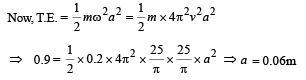Match the Following

DIRECTIONS (Q. No. 1-2) : Each question contains statements given in two columns, which have to be matched. The statements in Column-I are labelled A, B, C and D, while the statements in ColumnII are labelled p, q, r and s. Any given statement in Column-I can have correct matching with ONE OR MORE statement(s) in Column-II. The appropriate bubbles corresponding to the answers to these questions have to be darkened as illustrated in the following example :

If the correct matches are A-p, s and t; B-q and r; C-p and q; and D-s then the correct darkening of bubbles will look like the given.

Q.1. Column I describes some situations in which a small object moves. Column II describes some characteristics of these motions.

Match the situations in Column I with the characteristics in Column II and indicate your answer by darkening appropriate bubbles in 4 × 4 matrix given in the ORS.

 Column I Column II (A) The object moves on the x -axis under a conservative force in such a way that its ''speed " and position'' satisfy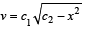where c1 and c2 are positive constants. (p)   The object executes a simple harmonic motion. (B) The object moves on the x- axis in such a way that its velocity and its displacement from the origin satisfy v = – kx, where k is a positive constant. (q)   The object does not change its direction. (C) The object is attached to one end of a mass-less spring of a given spring constant. The otherend of  the spring is attached to the ceiling of  an elevator.  Initially everything is at rest. The elevator starts going upwards with a constant acceleration a. The motion of the object is observed from the elevator during the period it maintains this  acceleration. (r)  The kinetic energy of the object keeps on decreasing. (D) The object is projected from the earth's surface vertically upwards with a  speed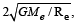where, Me is  the mass of the earth and Re is the radius of the earth, Neglect forces from objects other than the earth. (s)  The object can change its direction only once.

Ans. (A) → p; (B) → q, r; (C) → p; (D) → q, r

Solution. A → p

Reason : For a simple harmonic motion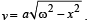On comparing it with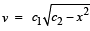we find the two comparable.

B q, r

Reason : v = – k x when x is positive; v is  – ve, and as x decreases, v decreases.

Therefore kinetic energy will decreases. When x = 0, v = 0.

Therefore the object does not change its direction.

When x is negative, v is positive. But as x decreases in magnitude, v also decreases. Therefore kinetic energy decreases. When x = 0, v = 0. Therefore the object does not change its direction.

C    p

Reason : When a = 0, let the spring have an extension x.

Then k x = mg.

When the elevator starts going upwards with a constant acceleration, as seen by the observer in the elevator, the object is at rest.

∴ ma + mg = k x'

⇒ ma = k (x' – x)     (Since a is constant)

D q, r The speed is √2 times the escape speed. Therefore the object will leave the earth. It will therefore not change the direction and its kinetic energy will keep on decreasing.

Q.2. Column I gives a list of possible set of parameters measured in some experiments. The variations of the parameters in the form of graphs are shown in Column II. Match the set of parameters given in Column I with the graph given in Column II. Indicate your answer by darkening the appropriate bubbles of the 4 × 4 matrix given in the ORS.

 Column I Column II (A) Potential energy of a simple pendulum (y axis) as a function of displacement (x axis)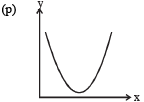(B) Displacement (y axis) as a function of time (x axis) for a one dimensional motion at zero or constant acceleration when the body is moving along the positive x-direction.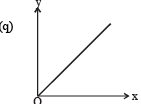(C) Range of a projectile (y axis) as a function of its velocity (x axis) when projected at a fixed angle.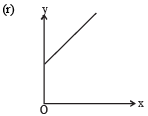(D) The square of the time period (y axis) of a simple pendulum as a function of its length (x axis)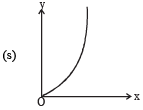Ans. (A) → p; (B) → q, r, s; (C) → s; (D) → q

Solution. A → p When simple pendulum is displaced from the mean position towards any of the extreme position, its potential energy increases. In case of a S.H.M. we get a parabola for potential energy versus displacement

B → q, r, s S = ut for zero acceleration. Therefore we get a straight line passing through the origin. Therefore option (q) is correct.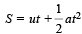for constant positive acceleration. In this case we get a part of parabola as a graph line between s versus t as shown by graph (s).

(p) is ruled out because if a is –ve and v is positive.

C → s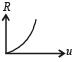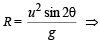R ∞ u2  (for consant θ and g)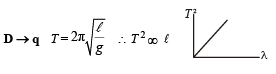The document Fill in the Blanks: Simple Harmonic Motion (Oscillations) | JEE Advanced Notes | Study Physics 35 Years JEE Main & Advanced Past year Papers - JEE is a part of the JEE Course Physics 35 Years JEE Main & Advanced Past year Papers.
All you need of JEE at this link: JEEUse Code STAYHOME200 and get INR 200 additional OFF Use Coupon Code

Top Courses for JEEPhysics 35 Years JEE Main & Advanced Past year Papers

88 docs|49 tests

Top Courses for JEETrack your progress, build streaks, highlight & save important lessons and more!

,

,

,

,

,

,

,

,

,

,

,

,

,

,

,

,

,

,

,

,

,

;